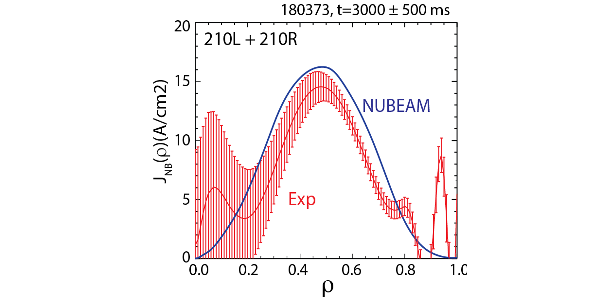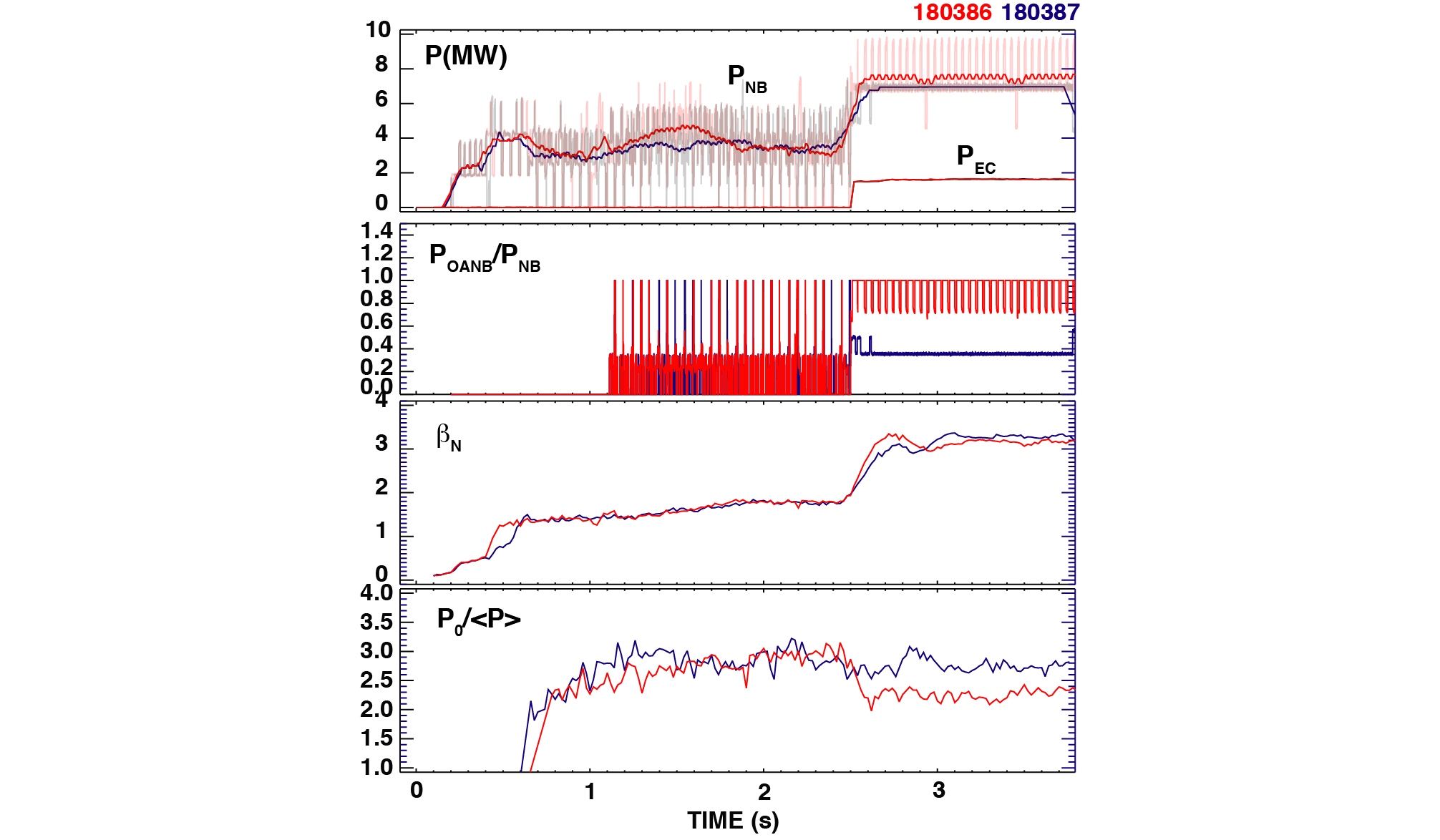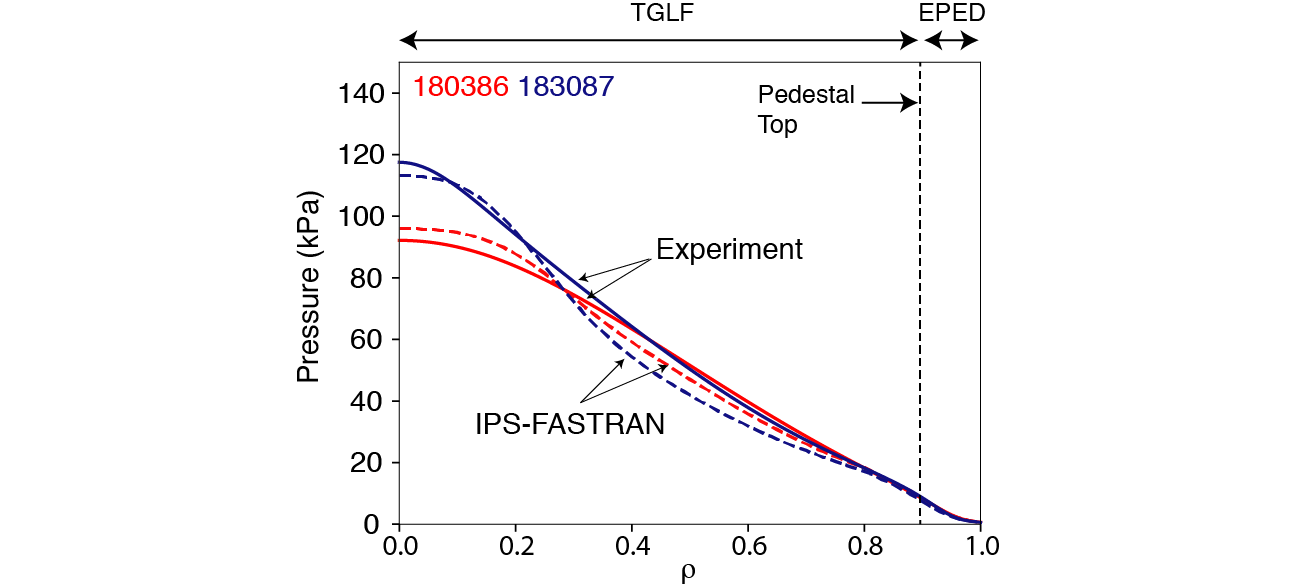Since 18 of December 2019 conferences.iaea.org uses Nucleus credentials. Visit our help pages for information on how to Register and Sign-in using Nucleus.

# 28th IAEA Fusion Energy Conference (FEC 2020)

May 10 – 15, 2021
Virtual Event
Europe/Vienna timezone
The Conference will be held virtually from 10-15 May 2021

## Off-axis Neutral Beam Current Drive for Advanced Tokamak

May 11, 2021, 8:30 AM
4h
Virtual Event

#### Virtual Event

Regular Poster Magnetic Fusion Experiments

### Speaker

Jin Myung Park (Oak Ridge National Laboratory)

### Description

Off-axis Neutral Beam Current Drive (NBCD) physics has been tested on DIII-D for Advanced Tokamak (AT) operation with increased off-axis injection power ($P_{OANB}\simeq7$ MW) by using the newly available, toroidally steerable co/counter off-axis neutral beam (CCOANB) injection capability. DIII-D experiments confirm that the new CCOANB drives current as predicted by the classical model NUBEAM for MHD quiescent plasmas (Fig. 1). Compared to on-axis injection, substantial broadening of the current and pressure profiles has been achieved with dominant OANB heating by injecting both the new CCOANB and the previous vertically steerable OANB. This is consistent with predictions of the theory-based IPS-FASTRAN integrated modeling that has guided the DIII-D beam system upgrade for the development of reactor relevant $\beta_N>4$ steady-state scenarios. Projecting to the Compact Advanced Tokamak (CAT) fusion pilot plant shows that off-axis NBCD aligns well with the high bootstrap current $f_{BS}>0.8$ operation, maintaining a broad current profile with $q_{min}>2$ and excellent off-axis NBCD efficiency.The NBCD profile driven by the new CCOANB was measured in H-mode plasmas and compared with modeling. The NBCD measurement$^1$ is based on the local measurement of the magnetic field pitch angles from Motional Stark Effect (MSE) diagnostics. These pitch angles are converted to the flux-surface-average current density ($J_\parallel$) and parallel electric field ($E_\parallel$), either using kinetic EFIT equilibrium reconstruction or a more direct MSE analysis. This allows the beam driven current to be determined by $J_{NB} = J_\parallel – \sigma_{NEO}E_\parallel -J_{BS}$, where $\sigma_{NEO}$ and $J_{BS}$ are the neoclassical conductivity and bootstrap current density calculated by the Sauter model using the measured kinetic profiles as input. Differential NBCD measurement reduces model dependencies ($\sigma_{NEO}$ , $J_{BS}$) and systematic uncertainties of measurements. In this study, we compare two discharges, either i) on- and off-axis or ii) “Left” (more tangent) and “Right” (more perpendicular) off-axis NBCD in otherwise similar discharge conditions. The measured NBCD profiles driven by the new CCOANB in the co-current direction agree reasonably well with classical model of Monte Carlo beam ion slowing down calculation NUBEAM (Fig. 1). The NUBEAM modeling employs an accurate beam injection model validated against fast visible image and neutron measurement$^2$. The toroidal field direction (+BT) was chosen for better alignment of NBI to the local B, leading to good off-axis NBCD efficiency. The minimum value of q was maintained at $q_{min}>1$ without any significant core MHD activities. The measured $J_{NB}(\rho)$ in Fig. 1 shows a clear hollow NBCD profile with the peak at about half the minor radius $\rho\sim0.5$. The net driven current normalized to the total injection power is $I_{NB}/P_{NB} = 14.9$ kA/MW, which is as good as on-axis NBCD since the increased fraction of trapped electrons reduces the electron shielding in the outer radius region. The measured NBCD ($I_NB$) increases with the off-axis NB power. Higher NB power operation produced mild low-frequency MHD modes (n=2 and 3), resulting in the reduced NBCD compared with the classical model prediction. The measured NBCD profiles at the highest inject power matches the NUBEAM modeling with a modest anomalous beam ion diffusion $D_b = 0.3 ~{\rm m^2/s}$. However, even including the effect of finite $D_b$, the measured NBCD efficiency ($I_{NB}/P_{NB}$) does not decrease with $P_{NB}$.

A low pressure peaking factor has been obtained with dominant off-axis NB heating. Figure 2 compares two discharges with different ratio of the OANB power to the total NB power ($f_{OANB} = P_{OANB}/P_{NB}$) at ~same injection power for elevated $q_{min} > {\sim1.5}$ discharges. The pressure peaking, $f_p = p_0/\langle p \rangle$ decreases substantially when the on-axis NB power (blue traces) is replaced by the new CCOANB (red traces), while maintaining $\beta_N\sim3$ with high total ($H_{89}\sim2.3$) and thermal ($H_{98}\sim1.25$) energy confinement. The lower pressure peaking also results in the increased low-n ideal $\beta_N$ stability limit$^3$. Both discharges inject full available power from the vertically steerable OANB at the maximum tilt angle. This profile broadening has been reproduced by the IPS-FASTRAN modeling$^4$ that integrates theory-based models of core transport (TGLF), edge pedestal (EPED1), equilibrium (EFIT), stability (DCON), heating and current drive (NUBEAM, TORAY) self-consistently to find steady-state ($d/dt = 0$) solutions. Figure 3 compares the pressure profile between the measurement (kinetic EFIT equilibrium reconstruction) and the IPS-FASTRAN modeling, where all transport channels ($n_e$, $T_e$, $T_i$, rotation, and current) are predicted without any significant free input parameters except the density and rotation values at the pedestal top.The measured off-axis NBCD does not lose CD efficiency by going to a larger radius, which is beneficial for future AT reactors. The IPS-FASTRAN modeling predicts significant improvement of energy confinement time for broad current profile with flat or weak negative magnetic shear, compared with monotonic $q_0\sim1$, for the AT reactors. Projecting to the CAT $^5$ Fusion Pilot Plant with R = 4 m, B = 7 T shows an excellent off-axis NBCD efficiency for high Greenwald density fraction operation leading to high fusion performance. Off-axis NBCD aligns well with the high bootstrap current $f_{BS}>0.8$ operation maintaining broad current profile with $q_{min}$>2.This material is based upon work supported by the U.S. Department of Energy, Office of Science, Office of Fusion Energy Sciences, using the DIII-D National Fusion Facility, a DOE Office of Science user facility, under Awards DE-AC05-00OR22725, DE-FC02-04ER54698, DE-AC02-09CH11466, DE-FG02-07ER54917, DE-SC0012656

$^1$ J.M. Park, et al., Phys. Plasmas 16, 092508 (2009).
$^2$ B.A. Grierson, et al., submitted to this conference.
$^3$ B.S. Victor, et al., submitted to this conference.
$^4$ J.M. Park, et al., Phys. Plasmas 25, 012506 (2018).
$^5$ R.J. Buttery, et.al., IAEA FEC, FIP-P3/26 (2018).

Affiliation Oak Ridge National Laboratory United States

### Primary authors

Jin Myung Park (Oak Ridge National Laboratory) C. Craig Petty (General Atomics) Michael Van Zeeland (General Atomics) B.A. Grierson (Princeton Plasma Physics Laboratory) William W. Heidbrink (University of California Irvine) Cami Collins (General Atomics) Brian Victor (Lawrence Livermore National Laboratory) Dr Kathreen Thome (General Atomics) Christopher T. Holcomb (Lawrence Livermore National Laboratory) Kyungjin Kim (Oak Ridge National Laboratory)